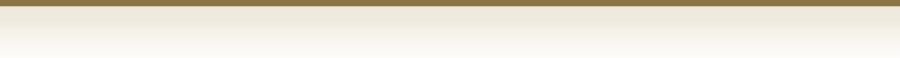1. Can PWTK be used on HPC clusters, i.e., does it support batch queuing?
Yes. PWTK makes submitting jobs to batch queues easier for the user. See the corresponding section in the tutorial and also the info provided here.
2. Can PWTK use the Quantum ESPRESSO executables from the container?
Yes, but it requires specific instructions. Here is an example how to use Quantum ESPRESSO executables from the Singularity container:
prefix "singularity exec /path/to/container.simg mpirun -np N"
serial_prefix "singularity exec /path/to/container.simg"
bin_query false
where /path/to/container.simg is the full pathname of the singularity container with Quantum ESPRESSO executables and N stands for the number of processors. To make these instructions permanent, these specs can be included in the main user configuration file ~/.pwtk/pwtk.tcl.
3. I want to grep specific data from the pw.x output file, but PWTK does not have the corresponding retrieval function?
PWTK has kind of "grep" and "egrep" functions (::pwtk::grep, ::pwtk::grep_field, ::pwtk::egrep, ::pwtk::egrep_field). For example, suppose that we want to retrieve the following lines from a pw.x output file:
internal energy E=F+TS = ............ Ry
where dots stand for a given internal energy value. This can be achieved by:
::pwtk::grep "internal energy E=F+T" file.out
where file.out is the name of the output file. To grep the last such line from the output:
::pwtk::grep "internal energy E=F+T" file.out end
Only the energy values can be obtained by:
::pwtk::grep_field "internal energy E=F+T" file.out 4
where 4 is the index of field to retrieve. Beware that counting starts from 0, hence 4 means 5th field. To retrieve the last internal energy from the output file:
::pwtk::grep_field "internal energy E=F+T" file.out 4 end
4. Can PWTK read Quantum ESPRESSO input files, i.e., can it utilize the already existing input files?
Yes. There are two different ways how to deal with this issue:
1. To utilize Quantum ESPRESSO input files directly without loading them into PWTK. This works for almost any Quantum ESPRESSO executable. Here is an example how to run a calculation with an existing input file within the PWTK script:
run code.x file.in
where code.x stand for the Quantum ESPRESSO executable (e.g. pw.x, neb.x) and file.in is the name of the input file. The output is then written to file.out. For more details, see ::pwtk::run.
2. To load the existing Quantum ESPRESSO input file into PWTK. This works only for explicitly supported codes, which are listed here. Here is an example for pw.x: